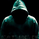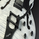จำนวนเข้าชม 55139
For both scripts - Go to 'MAKE IT MINE'
Modified scripts for Binary trading 1- 3 min charts / 5 -15 min intervals
vdubus BinaryPro 1
Vdubus BinaryPro 2
------------------------------
Vdubus BinaryPro Money Management Strategy: $10 -$50,000 in 15 trades
Tip * break consecutive trades down to lots of 5 compounded / repeat to build up a solid equity foundation., then move on the the next 5 x 3 = 15
if you lose one trade out of the 5, restart the proses. You only ever lose the initial trade size you started with * DOUBLE YOUR WINNINGS NOT YOUR LOSSES !
If you struggle to win 5 compounded trades in a row, compound 2 or 3 trades at a time
//Script editor vdubus
//vdubong 20 (set variables as required Upper & Lower)
study(title="vdubus BinaryPro 1", shorttitle="vdubus BinaryPro 1", overlay=true)

length1 = input(20, minval=1, title="Upper Channel")
length2 = input(20, minval=1, title="Lower Channel")

upper = highest(length1)
lower = lowest(length2)
basis = avg(upper, lower)

l = plot(lower, style=circles, linewidth=2, color=fuchsia, title="lower")
u = plot(upper, style=circles, linewidth=2, color=fuchsia, title="upper")

//-----------------Built in MA50-----------------------------------------
m1_src = close
m1_p = input(50, title="MA1 Period:")

plot(sma(m1_src, m1_p), color=red, linewidth=2, title="MA1")

//-----------------Built in BB20-------------------------------------------
bb1_src = close
bb1_l = input(20, minval=1), bb1_mult = input(1.5, minval=0.001, maxval=400)
bb1_dev = bb1_mult * stdev(bb1_src, bb1_l)
bb1_upper = basis + bb1_dev
bb1_lower = basis - bb1_dev
bb1_p1 = plot(bb1_upper, color=blue)
bb1_p2 = plot(bb1_lower, color=blue)
fill(bb1_p1, bb1_p2, transp=90)
//-----------------Built in BB50 -----------------------------------------
//bb2_src = close
//bb2_l = input(50, minval=1), bb2_mult = input(1.5, minval=0.001, maxval=400)
//bb2_dev = bb2_mult * stdev(bb2_src, bb2_l)
//bb2_upper = basis + bb2_dev
//bb2_lower = basis - bb2_dev
//bb2_p1 = plot(bb2_upper, color=blue)
//bb2_p2 = plot(bb2_lower, color=blue)
//fill(bb2_p1, bb2_p2, transp=90)


## ความคิดเห็น